# LeetCode周賽311，中規中矩的裸題大賽……## 最小偶倍數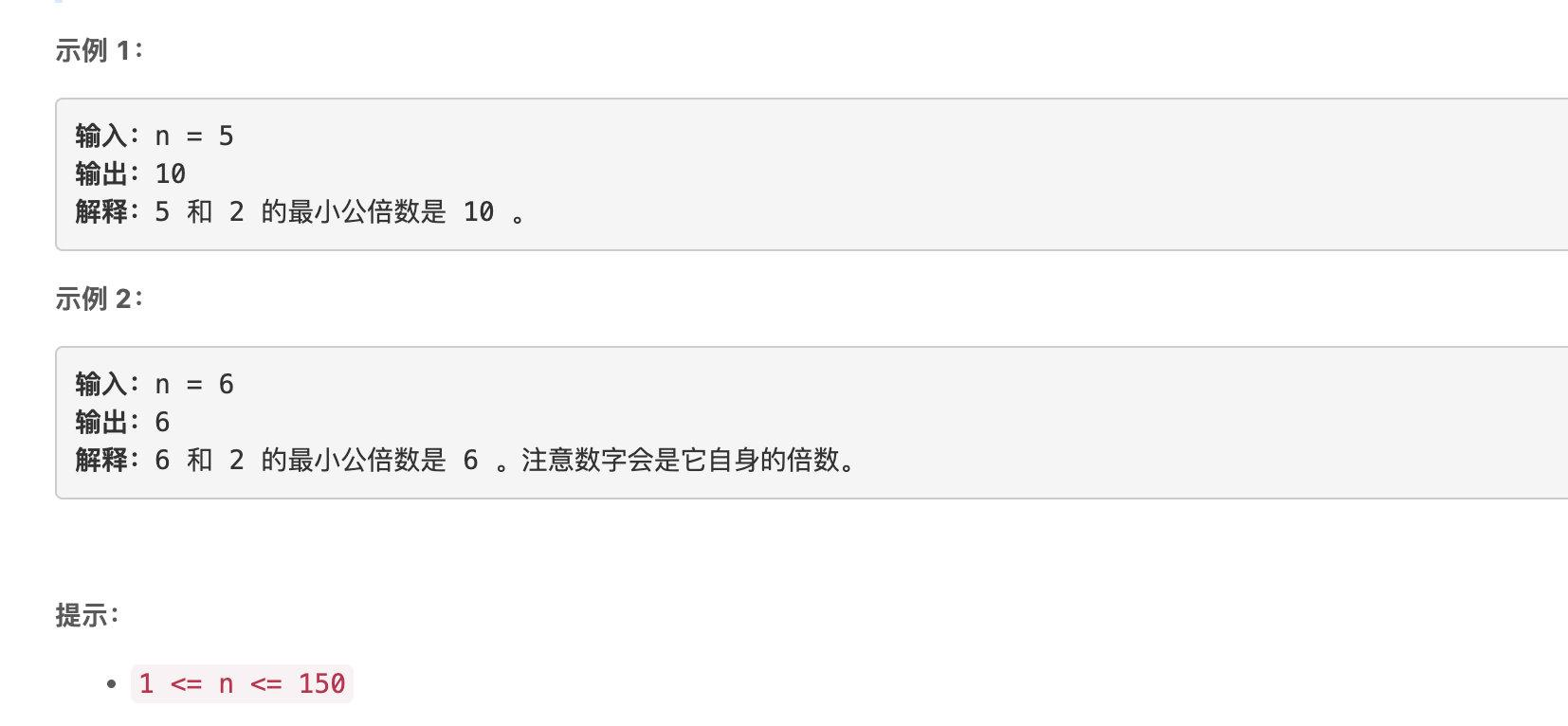### 題解

```cpp class Solution { public: int smallestEvenMultiple(int n) { return n % 2 ? n << 1: n; } };```

## 最長的字母序連續子字串的長度

• 例如，`"abc"` 是一個字母序連續字串，而 `"acb"``"za"` 不是。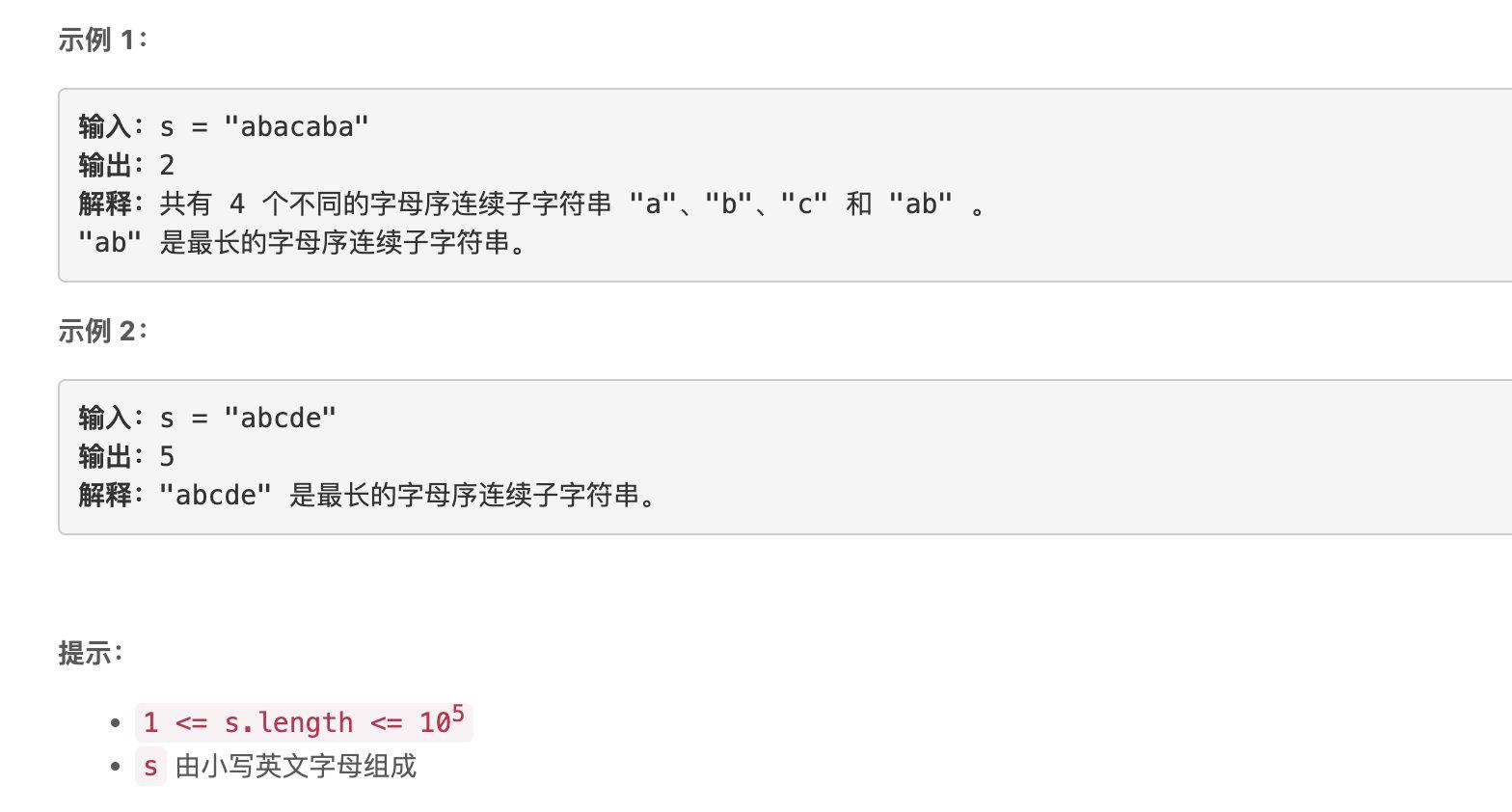### 題解

two pointer裸題。

```cpp class Solution { public: int longestContinuousSubstring(string s) { int l = 0;

``````    int ret = 1;
for (int r = 1; r < s.length(); r++) {
if (s[r] != s[r-1]+1) {
l = r;
}
ret = max(r - l + 1, ret);
}
return ret;
}
``````

}; ```

## 反轉二叉樹的奇數層

• 例如，假設第 3 層的節點值是 `[2,1,3,4,7,11,29,18]` ，那麼反轉後它應該變成 `[18,29,11,7,4,3,1,2]`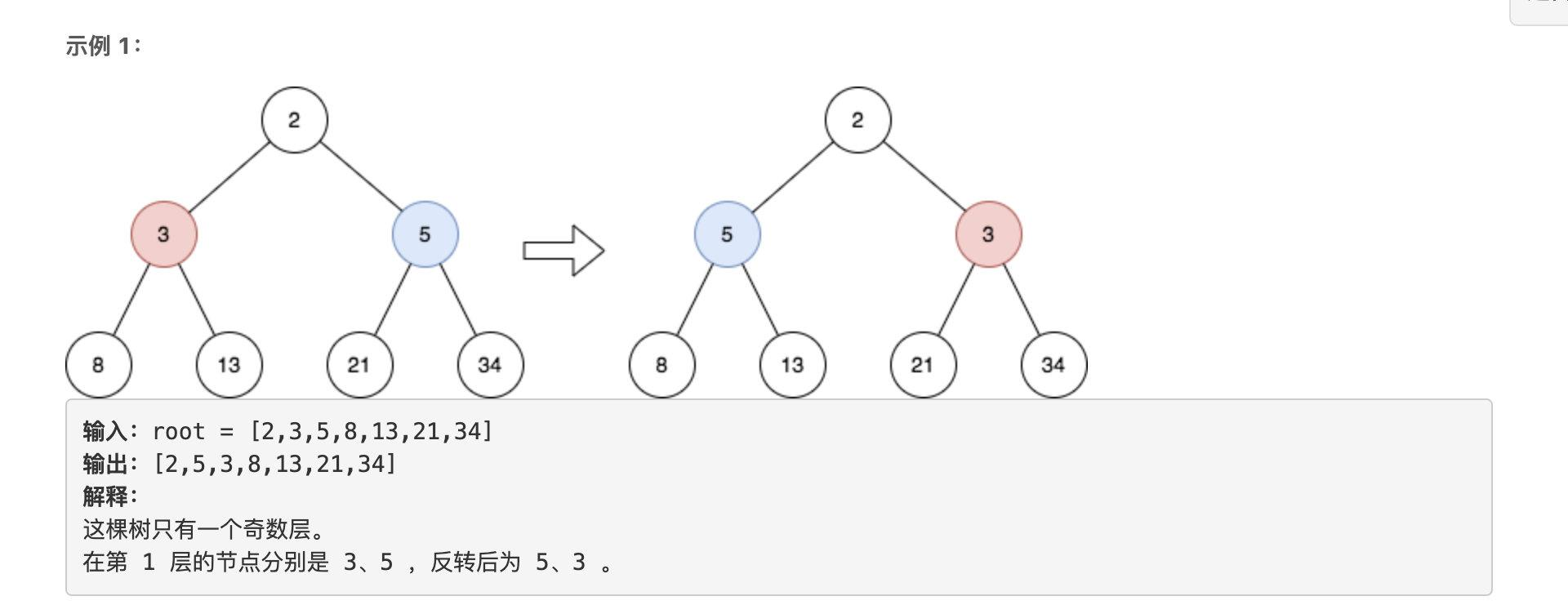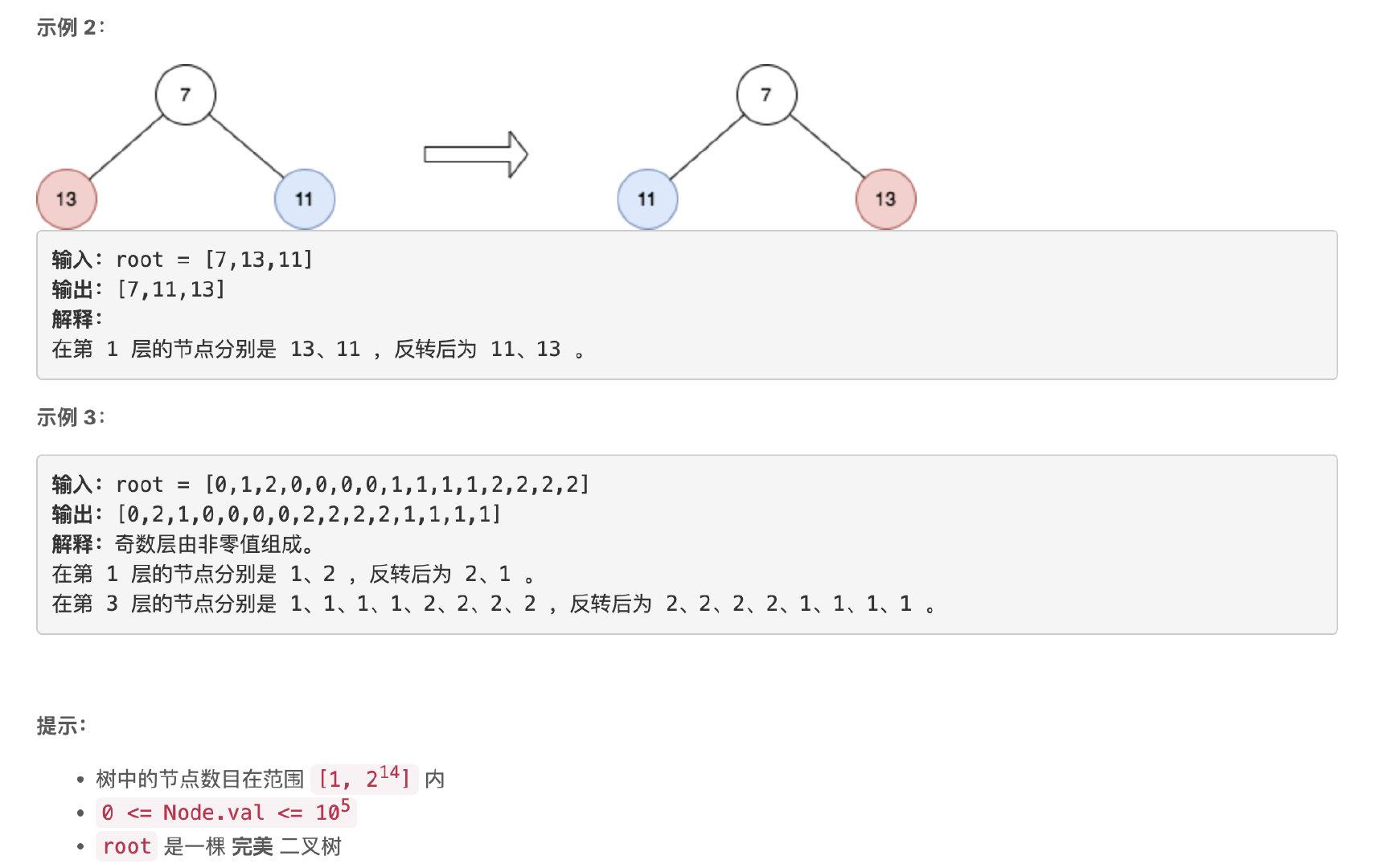### 題解

```cpp / * Definition for a binary tree node. * struct TreeNode { * int val; * TreeNode left; * TreeNode right; * TreeNode() : val(0), left(nullptr), right(nullptr) {} * TreeNode(int x) : val(x), left(nullptr), right(nullptr) {} * TreeNode(int x, TreeNode left, TreeNode right) : val(x), left(left), right(right) {} * }; / class Solution { public: TreeNode reverseOddLevels(TreeNode* root) { // cur儲存當前層的節點，next儲存下一層 vector cur, next; // 記錄下一層是否要翻轉 bool flag = true;

``````    cur.push_back(root);
while (!cur.empty()) {
for (auto &u: cur) {
if (u->left != nullptr) {
next.push_back(u->left);
next.push_back(u->right);
}
}

if (flag) {
int l = 0, r = next.size() - 1;
while (l < r) {
swap(next[l]->val, next[r]->val);
l++;
r--;
}
}
flag = !flag;
cur = next;
next = vector<TreeNode*> ();
}
return root;
}
``````

}; ```

## 字串的字首分數和

• 例如，如果 `words = ["a", "ab", "abc", "cab"]` ，那麼 `"ab"` 的分數是 `2` ，因為 `"ab"``"ab"``"abc"` 的一個字首。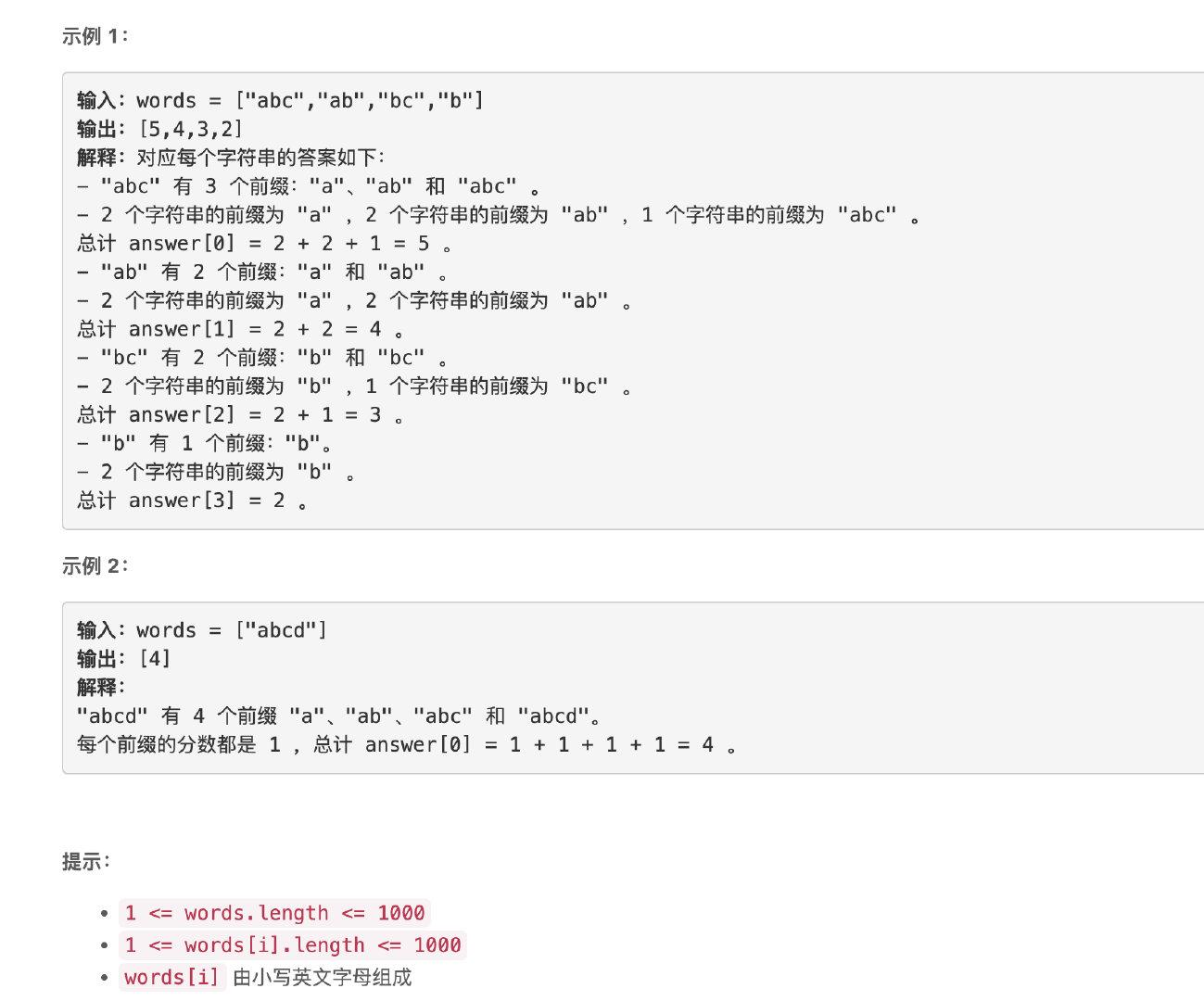### 題解

```cpp class Solution { public:

``````struct Node {
int v;
Node* chs = {nullptr};
Node(): v(1) {};
};

struct Trie {
Node *node;
Trie() {
node = new Node();
}
void insert(string &str) {
Node *cur = node;
for (auto c: str) {
int idx = c - 'a';
if (cur->chs[idx] == nullptr) {
cur->chs[idx] = new Node();
}else {
cur->chs[idx]->v++;
}
cur = cur->chs[idx];
}
}
int query(string &str) {
int ret = 0;
Node *cur = node;
for (auto c: str) {
int idx = c - 'a';
if (cur->chs[idx] == nullptr) break;
ret += cur->chs[idx]->v;
cur = cur->chs[idx];
}
return ret;
}
};

vector<int> sumPrefixScores(vector<string>& words) {
vector<int> ret;
Trie* t = new Trie();
for (auto &st : words) {
t->insert(st);
}

for (auto &st: words) {
int cur = t->query(st);
ret.push_back(cur);
}
return ret;
}
``````

}; ```